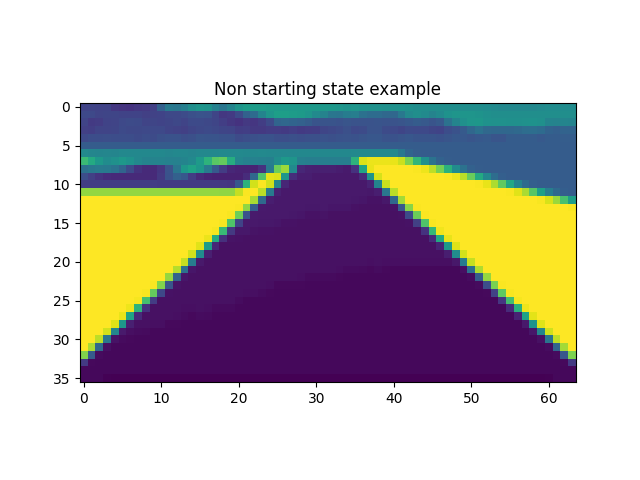# Grayscale image plotted to have colours

I am using PIL first to convert a saved image to grayscale then resize and convert to a tensor object using the following code:

``````gray_image = ImageOps.grayscale(img)

resize = T.Compose([

T.Resize((36,64))  # height, width

,T.ToTensor()

])
``````

However, when i plot it using:

``````plt.figure()
plt.imshow(resize(gray_image).squeeze(0).permute(1, 2, 0).cpu(), interpolation='none')
plt.title('Non starting state example')
plt.show()
``````

it shows me a plot like below that is not a grayscale.Is it because of the way the tensor is plotted?

`matplotlib` uses the default colormap if you don’t specify another one. Use `plt.imshow(..., cmap='gray')` to use the grayscale colormap.

Thank you for your reply. Wouldn’t that be like manually setting the colors to be in grayscale? I want to see the tensor image as it is, which is supposed to be grayscaled. Not sure if that make sense.

If your tensor doesn’t contain a color channel dimension the colormap is undefined and matplotlib will use its default one.
In case the tensor represents a real grayscale image, it would have a color channel size of 3 where all channels are equal.
This code shows the behavior:

``````x = torch.randint(0, 256, (100, 100, 1)).byte()
plt.imshow(x.numpy())

# create grayscale image
x = torch.cat((x, x, x), dim=2)
plt.imshow(x.numpy())
``````

While single-channel images could be considered grayscale, matplotlib treats them as a matrix.